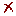Conversion

Mass Concentration

 From ToMemo kg/m3 kg/m3 g/m3 g/m3 mg/m3 mg/m3 µg/m3 µg/m3 kg/L kg/L g/L g/L g/dL g/dL mg/L mg/L mg/dL mg/dL mg/mL mg/mL µg/L µg/L µg/dL µg/dL µg/mL µg/mL ng/dL ng/dL ng/mL ng/mL gr/gal (US) gr/gal (US) gr/gal (UK) gr/gal (UK) lb/gal (US) lb/gal (US) lb/gal (UK) lb/gal (UK) lb/ft3 lb/ft3 ppm (1) ppm (1) Density of the Solution (ρ) =   kg/m3  (g/L) To Memory 01 Memory 02 Memory 03 Memory 04 Memory 05 Memory 06 Replacing Adding Subtracting Multiplying Dividing

Note 1:

Parts per million (ppm) is a dimensionless notation and, therefore, can only be used as a unit of measure of concentration, equivalent to mg/L, in aqueous solution of low concentration, with density equal to that of water (1000 kg/m3), since in this case one liter of solution has a mass equal to 1000 grams, being 1 mg/L = 1 mg/1000 g = 1 g/1000000 g = 1 ppm. For conversion of ppm to mass/volume concentration units, in solutions with different densities from 1000 kg/m3, fill the calculator field with the solution density value. For more information, see article from Wikipedia.

### Configurations

Do not use the number format settings
Maximum of decimal places

Accuracy of significant figures

Decimal separator

Thousands separator

Number Notation

Exponent Notation

Notation example:
12345.6 ou 1.23456e+4

Impression Page Title (Optional)